MCAT Physical : Physical Chemistry

Example Questions

← Previous 1 3 4 5 6 7 8 9 19 20

Example Question #1 : Physical Chemistry

In an electrolytic cell, oxidation takes place at the __________.

Oxidation does not take place in an electrolytic cell.

anode

salt bridge

cathode

anode

Explanation:

Electrolytic cells have a negative electromotive force and require an outside energy source to power a non-spontaneous reaction. Galvanic cells, in contrast, have positive potentials and facilitate spontaneous reactions without the need of a power source.

Regardless of cell type, however, oxidation always takes place at the anode and reduction always takes place at the cathode. The flow of electrons is always from the anode to cathode.

Example Question #2 : Physical Chemistry

A student conducts an experiment for a chemistry class. The student wishes to explore power generation from different types of voltaic cells. He sets up three different cells, and then compares the amount of energy generated from each one.

One of his cells is shown below as an example. Both remaining cells follow the same layout.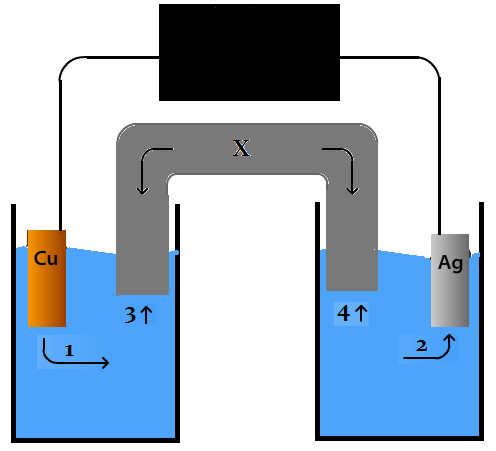How would the voltaic cell depicted above differ from an electrolytic cell?

An electrolytic cell produces energy based on differences in concentrations between the half cells

An electrolytic cell requires energy input

An voltaic cell requires energy input to begin the reduction process

An electrolytic cell produces more energy

An electrolytic cell produces more energy, but only after an input of energy to begin the process

An electrolytic cell requires energy input

Explanation:

Electrolytic cells require an input of energy, and are used to plate metals by functionally running a voltaic cell in reverse.

Example Question #3 : Physical Chemistry

Which of the following is not true of electrolytic cells?

Electricity is conducted by the motion of ions

Electrons travel toward the cathode

Oxidation occurs at the anode

No battery is required for electrolysis to take place

The cell potential is negative

No battery is required for electrolysis to take place

Explanation:

For electrolytic cells, the cell potential is negative; in contrast, galvanic/voltaic cells have positive cell potentials. Electrolysis reactions can only occur if the total potential is positive. An additional voltage input, such as a battery, is required so that the sum of potentials in the electrolytic cell is greater than zero.

Oxidation always occurs at the anode, regardless of cell type, and electrons always travel toward the site of reduction (the cathode). In a galvanic cell, the cathode is positively charged, naturally drawing the flow of electrons. In an electrolytic cell, the cathode is negatively charged, but still requires the flow of electrons to allow reduction to occur. An induced current from a battery is used to propel these electrons against their natural flow.

Example Question #4 : Physical Chemistry

Which of the following is true about electrolysis?

I. It only involves reactions with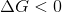II. It requires a voltage source

III. It is an exothermic process

I and II

II and III

I only

II only

II only

Explanation:

Electrolysis is a specific type of reaction that occurs in an electrolytic cell. An electrochemical cell contains an anode and a cathode that facilitate a redox reaction. In an electrolytic cell (a type of electrochemical cell) the redox reaction that is carried out is a nonspontaneous reaction. Recall that the change in Gibbs free energy for a nonspontaneous, or unfavorable, reaction is always positive; therefore, for electrolysis in an electrolytic cell, the redox reaction has a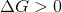. Statement I is false.

Nonspontaneous reactions are reactions that are unfavorable. This means that energy is required to carry out the reaction. In an electrolytic cell, energy is provided in the form of voltage input. The voltage provided pushes the reaction in the unfavorable direction; therefore, electrolysis reactions require a voltage source. Statement II is true.

Since it requires energy, an electrolysis reaction is considered to be an endothermic process. Recall that endothermic processes are reactions that take in (or require) energy, whereas exothermic processes are reactions that release energy; therefore, electrolysis is an endothermic process. Statement III is false.

Example Question #5 : Physical Chemistry

Consider the following reaction: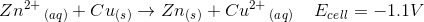How much voltage will you have to apply to carry out this reaction?

No voltage needs to be applied because this reaction represents an electrolytic cell

Greater than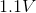No voltage needs to be applied because this reaction represents a galvanic cell

Less thanGreater thanExplanation:

The question states that the reaction has a negative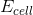; therefore, the reaction is nonspontaneous. Nonspontaneous reactions are carried out in electrolytic cells (as opposed to galvanic cells). A reaction usually proceeds in the spontaneous direction; therefore, to carry out nonspontaneous reactions you must put energy into the system. Without energy, the reaction shown will occur in the reverse direction.

In an electrolytic cell, energy is provided by an external voltage source. Without energy, the electrolytic cell will have a voltage ofand the spontaneous (reverse) reaction will occur. For the nonspontaneous reaction to occur, you must attach a voltage source in such a way that the voltage applied is greater thanand is applied in the opposite direction (nonspontaneous reaction direction). This will force the reaction in the reverse direction and the nonspontaneous reaction will occur; therefore, the external voltage source must provide a voltage greater than.

Example Question #6 : Physical Chemistry

A cubical block has a mass of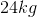and a density of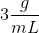. Which of the following values most closely approximates the height of the block?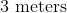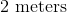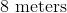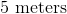Explanation:

To solve this question, you need to know the definition of density: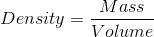The question gives us the mass of the block and the density of the block. We can use these values to solve for the volume of the block.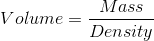Density has to be converted from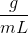to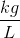(SI units). Recall that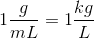; therefore,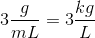.

Solving for volume of the block gives us: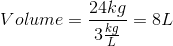A liter is defined as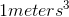; therefore,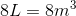.

Remember that the volume of a cube is calculated as follows: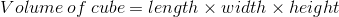Since the cube has the same length, width, and height, the equation above can be rewritten in terms of height as follows: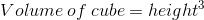This means that the height of the cube can be calculated by taking the cube root of volume: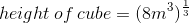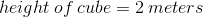The length, the width, and the height of this cubical block is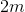.

Example Question #7 : Physical Chemistry

Which of the following is true regarding the density of solids?

Solids usually have greater density than liquids because they have larger volumes

Density of a solid can be calculated by dividing the weight of the solid by the volume of the solid

A sample of a substance in its solid phase will have the same mass as the same sample in its liquid phase

Solid water has a higher density than liquid water

A sample of a substance in its solid phase will have the same mass as the same sample in its liquid phase

Explanation:

Density is defined as mass divided by volume. Recall that the solid phase is usually characterized by tightly packed molecules. As a result, solids usually take up less volume than liquids and, consequently, have greater densities than liquids.

The most common exception to this rule is water. Liquid water has a density of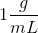, whereas solid water (ice) has a density of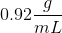.

Density of any substance (solid or liquid) is calculated as follows: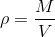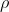is density of the substance,is mass, andis volume. The density of a substance does not depend on the weight of the object.

Changing a substance from its solid phase to its liquid phase only alters volume; it does not change the mass. Remember that the mass of a substance depends on the amount of molecules. Changing from solid to liquid will increase the spacing between the molecules (volume), but it will not change the amount of molecules (mass); therefore, the solid phase and the liquid phase of a sample of substance will have the same mass.

Example Question #8 : Physical Chemistry

What will happen to the density of iron if you increase the temperature to its melting point?

The density will decrease because the mass will decrease

The density will decrease because the volume will increase

The density will increase because the mass will increase

The density will increase because the volume will decrease

The density will decrease because the volume will increase

Explanation:

Increasing a substance’s temperature to its melting point will convert the substance from a solid to a liquid. This will increase the volume of the substance because the molecules within the substance will be farther apart from each other; however, the amount of molecules (mass of the substance) will remain unchanged.

Recall the definition of density:Since the volume increases and the mass stays the same, the density of iron will decrease when you change the temperature to its melting point.

Example Question #9 : Physical Chemistry

Consider the following factors:

I. Temperature

II. Pressure

Which of these two factors will alter the density of a substance?

Only II because it changes the mass of the substance

Only I because it changes the volume of the substance

Both I and II because I changes the volume and II changes the mass of the substance

Both I and II because both change the volume of the substance

Both I and II because both change the volume of the substance

Explanation:

Density is defined as follows:To alter density, we need to alter mass and/or volume. Remember that mass can only be altered by removing from or adding to the sample. Temperature and pressure do not change the amount of substance; therefore, they do not alter the mass of the substance.

Changing temperature will alter the distance between the molecules within the substance. Increasing the temperature will increase the distance between the molecules, which will increase the volume taken up by the substance. Similarly, decreasing the temperature will decrease the distance between the molecules, which will decrease the volume taken up by the substance. This means that changing temperature will alter the volume of the substance and, subsequently, the density of the substance.

Applying pressure also changes the distance between the molecules. Increasing the pressure will force the molecules to be closer together, whereas decreasing the pressure will enable the molecules to spread and separate from each other; therefore, pressure also alters density by changing the volume of the substance.

Example Question #10 : Physical Chemistry

Consider a horizontal tube that has a length of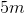and a diameter of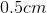. You stretch this tube by applying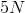of force on each end of the tube. What is happening to the tube when you are applying the force?

The tube is experiencing a stress of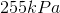and is undergoing tension

The tube is experiencing a stress of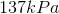and is undergoing compression

The tube is experiencing a stress ofand is undergoing compression

The tube is experiencing a stress ofand is undergoing tension

The tube is experiencing a stress ofand is undergoing tension

Explanation:

To answer this question, you need to solve for the stress experienced by the tube. Stress is defined as: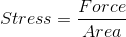Since this is a tube, the area we are concerned with is the circular cross-sectional area of the tube. The question states that the diameter is. This means that the radius (which is half the length of the diameter) is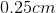. Recall that meters is the SI unit for distance; therefore, we need to convert the radius to meters: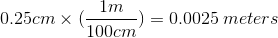This means that the radius of the tube, in meters, is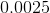. The area of a circle is: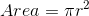Using this formula and our radius, we can find the cross-sectional area: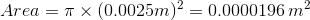The stress experienced by the tube is: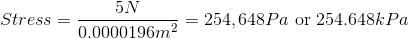The closest answer choice is.

The question states that the tube is being stretched by the force. This means that the tube is undergoing tension because you are pulling the tube and applying a force outwards. If the force was applied in the opposite direction (inwards), then the tube would be undergoing compression (the tube would shorten).

← Previous 1 3 4 5 6 7 8 9 19 20